# Prime avoidance lemma

View other indispensable lemmata

## Statement

Let$R$ be a commutative unital ring. Let$I_1, I_2, \ldots, I_n$ and$J$ be ideals of$R$, such that$J \subset \bigcup_j I_j$. Then, if$R$ contains an infinite field or if at most two of the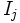$I_j$s are not prime, then$J$ is contained in one of the$I_j$s.

If$R$ is graded, and$J$ is generated by homogeneous elements of positive degree, then it suffices to assume that the homogeneous elements of$J$ are contained in$\bigcup_j I_j$. However, we need to add the further assumption that all the$I_j$s are prime.

## Importance

The prime avoidance lemma is useful for establishing dichotomies; in particular, if$J$ is an ideal which is not cintained in any of the$I_j$s, then$J$ has an element which is contained in none of the$I_j$s.

## Proof

### If the ring contains an infinite field

In this case, the proof boils down to two observations:

• Any ideal of the ring is also a vector space over the infinite field
• A vector space over an infinite field cannot be expressed as a union of finitely many proper subspaces

### If at most two of the ideals are not prime

The proof in this case proceeds by induction. The crucial ingredients to the proof are:

1. If two of the three elements$a,b,a+b$ belong to an ideal, so does the third (the fact that ideals are additive subgroups)
2. If, for a product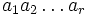$a_1a_2\ldots a_r$, any$a_i$ belongs to an ideal, so does the product
3. If a product$a_1a_2 \ldots a_r$ belongs to a prime ideal, then one of the$a_i$s also belongs to that prime ideal

We now describe the proof by induction. The case$n=1$ requires no proof; the case$n=2$ follows from observation 1 (in other words, we only need to use that both are additive subgroups). Namely, suppose$J$ is not a subset of either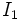$I_1$ or$I_2$. Pick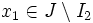$x_1 \in J \setminus I_2$ and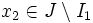$x_2 \in J \setminus I_1$. Clearly,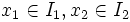$x_1 \in I_1, x_2 \in I_2$. Then$x_1 + x_2$ is in$J$, hence it must be inside$I_1$ or$I_2$. This contradicts observation 1. Further information: Union of two subgroups is not a subgroup

For$n > 2$, we use induction. Suppose, without loss of generality, that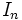$I_n$ is a prime ideal. Also assume without loss of generality that$J$ is not contained in the union of any proper subcollection of$I_1, I_2, \ldots, I_n$ (otherwise, induction applies). Thus, we can pick$x_i \in J \setminus \bigcup_{j \ne i} I_j$ for each$i$. Clearly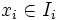$x_i \in I_i$.

We now consider the element: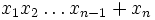$x_1x_2 \ldots x_{n-1} + x_n$

This is in$J$, hence it must be in one of the$I_i$s. We consider two cases:

• The sum is in$I_n$: Then, by observation 1,$x_1x_2 \ldots x_{n-1} \in I_n$. By observation 3,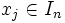$x_j \in I_n$ for some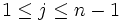$1 \le j \le n-1$, a contradiction.
• The sum is in$I_j$ for some$1 \le j \le n-1$: Observation 2 tells us that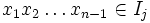$x_1x_2\ldots x_{n-1} \in I_j$, so observation 1 yields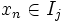$x_n \in I_j$, a contradiction

In this case, the proof is the same, except that we can now get started on the proof only after raising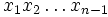$x_1x_2\ldots x_{n-1}$ and$x_n$ to positive powers so that the new terms have equal degrees, and can be added. In this case, we need all the$I_j$s to be prime to ensure that even after taking powers, the elements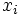$x_i$ still avoid the ideals$I_j$, for$j \ne i$.